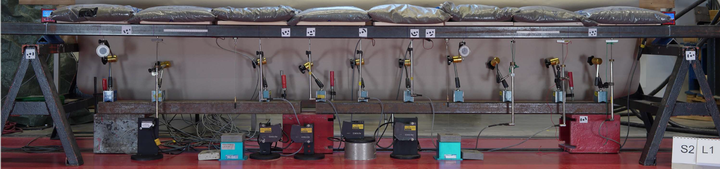# Physics-informed Gaussian process model for Euler-Bernoulli beam elements### Abstract

A physics-informed machine learning model, in the form of a multi-output Gaussian process, is formulated using the Euler-Bernoulli beam equation. Given appropriate datasets, the model can be used to regress the analytical value of the structure’s bending stiffness, interpolate responses, and make probabilistic inferences on latent physical quantities. The developed model is applied on a numerically simulated cantilever beam, where the regressed bending stiffness is evaluated and the influence measurement noise on the prediction quality is investigated. Further, the regressed probabilistic stiffness distribution is used in a structural health monitoring context, where the Mahalanobis distance is employed to reason about the possible location and extent of damage in the structural system. To validate the developed framework, an experiment is conducted and measured heterogeneous datasets are used to update the assumed analytical structural model.

Type
Publication
In Proceedings of IABSE Symposium – Challenges for Existing and Oncoming Structures, Prague, Czech Republic